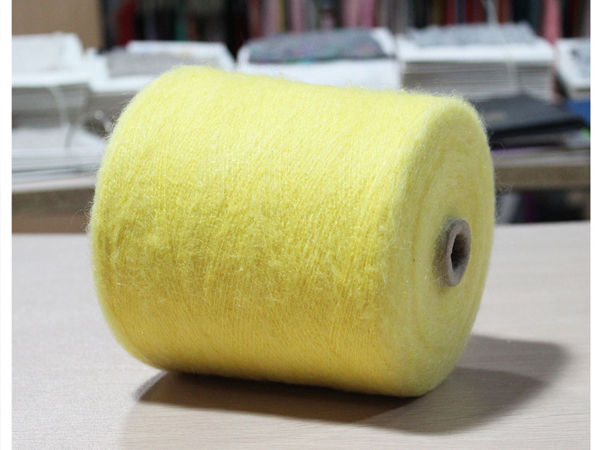﻿ 纱线支数的转换公式
HI,欢迎来到蓝美纺织网站

400-0823-488

# 纱线支数的转换公式

、定长制：
A. 特克斯：1000米长度的纱在公定回潮率时的质量克数称为特数。
公 式：Ntex =G/L×1000
式 中：G为纱的重量（克），L为纱的长度（米）
B. 旦尼尔：9000米长的丝在公定回潮率时的质量克数称为旦数。
公 式： Nden=G/L×9000
式 中：G为丝的重量（克），L为丝的长度（米）
、定重制：
A. 公支数（公支）：1克纱（丝）所具有的长度米数。
公 式：Nm=L/G
式 中：1为纱（丝）的长度（米），G为纱（丝）的重量（克）
B. 英支数（英支）：1磅纱线所具有的840码长度的个数。
公 式：S=L/（G×840）
式 中：L为纱（丝）的长度（码），G为纱（丝）的重量（磅）。
2
、单位换算
A
．特数Ntex与英制支数S
S=C/ Ntex
C为常数，化纤为590.5、棉纤为583，如果为混纺纱可根据混比进行计算，如：T/JC65/3545S纱线C=590.5*65%+583*35%=588，然后按公式计算便可）
B
．英制支数S与公制支数Nm

3
、特数Ntex 与公制Nm
Ntex ×Nm=1000
4
、特数Ntex 与旦数Nden
Nden=9*Ntex

`定长制计算公式：`
`(1)、旦尼尔(D) =g/L*9000 其中g为丝线的重量(克),L为丝线的长度(米)`
`(2)、特克斯(号数)[tex(H)]: tex=g/L*1000 其中g为纱(或丝)的重量(克),L为纱(或丝)的长度(米)`
`(3)、分特克斯(dtex): dtex=g/L*9000 其中g为丝线的重量(克),L为丝线的长度(米)`
`定重制计算公式:`
`(1)、公制支数(N):N=L/G 其中G为纱(或丝)的重量(克),L为纱(或丝)的长度(米)`
`(2)、英制支数(S):S=L/(G*840) 其中G为丝线的重量(磅),L为丝线的长度(码)`
`2、选择换算公式：`
`(1)、公制支数(N)与旦尼尔(D)的换算公式=9000/N`
`(2)、英制支数(S)与旦尼尔(D)的换算公式=5315/S`
`(3)、分特克斯(dtex)与特克斯(tex)的换算公式:1tex=10dtex`
`(4)、特克斯(tex)与旦尼尔(D)的换算公式:tex=D/9`
`(5)、特克斯(tex)与英制支数(S)的换算公式:tex=K/S K值:纯棉纱K=583.1 纯化纤K=590.5 涤棉纱K=587.6 棉粘纱(75:25)K=584.8 维棉纱(50:50)K=587.0`
`(6)、特克斯(tex)与公制数(N)的换算公式:tex=1000/N`
`(7)、分特克斯(dtex)与旦尼尔(D)的换算公式:dtex=10D/9`
`(8)、分特克斯(dtex)与英制支数(S)的换算公式: dtex=10K/S K值:纯棉纱K=583.1 纯化纤K=590.5 涤棉纱K=587.6 棉粘纱(75:25)K=584.8 维棉纱(50:50)K=587.0`
`(9)、分特克斯(dtex)与公制支数(N)的换算公式:dtex=10000/N`#### 您的浏览历史